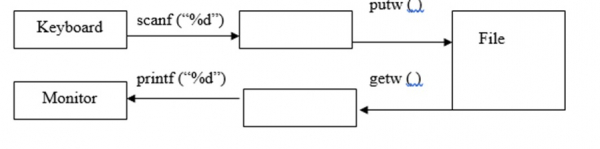# Explain the functions putw() and getw() in C language

CServer Side ProgrammingProgramming

#### C in Depth: The Complete C Programming Guide for Beginners

45 Lectures 4.5 hours

#### Practical C++: Learn C++ Basics Step by Step

Most Popular

50 Lectures 4.5 hours

#### Master C and Embedded C Programming- Learn as you go

66 Lectures 5.5 hours

File is collection of records or is a place on hard disk, where data is stored permanently.

## Operations on files

The operations on files in C programming language are as follows −

• Naming the file
• Opening the file
• Reading from the file
• Writing into the file
• Closing the file

## Syntax

The syntax for opening a file is as follows −

FILE *File pointer;

For example, FILE * fptr;

The syntax for naming a file is as follows −

File pointer = fopen ("File name", "mode");

For example,

fptr = fopen ("sample.txt", "r");
FILE *fp;
fp = fopen ("sample.txt", "w");

## putw( ) and getw( ) functions

putw( ) function is used for writing a number into file.

The syntax for putw() function is as follows −

## Syntax

putw (int num, FILE *fp);

For example,

## Example

FILE *fp;
int num;
putw(num, fp);

getw( ) function is used for reading a number from a file.

The syntax for getw() function is as follows −

## Syntax

int getw (FILE *fp);

For example,

## Example

FILE *fp;
int num;
num = getw(fp);The logic for writing numbers into a file is as follows −

fp = fopen ("num.txt", "w");
for (i =1; i<= 10; i++){
putw (i, fp);
}
fclose (fp);

The logic for reading numbers from a file is as follows −

fp =fopen ("num.txt", "r");
printf ("file content is\n");
for (i =1; i<= 10; i++){
i= getw(fp);
printf ("%d",i);
printf("\n");
}
fclose (fp);

## Program

Following is the C program for storing the numbers from 1 to 10 and to print the same −

Live Demo

#include<stdio.h>
int main( ){
FILE *fp;
int i;
fp = fopen ("num.txt", "w");
for (i =1; i<= 10; i++){
putw (i, fp);
}
fclose (fp);
fp =fopen ("num.txt", "r");
printf ("file content is\n");
for (i =1; i<= 10; i++){
i= getw(fp);
printf ("%d",i);
printf("\n");
}
fclose (fp);
return 0;
}

## Output

When the above program is executed, it produces the following result −

file content is
1
2
3
4
5
6
7
8
9
10